Operations with sets

Designation of sets and their elements. Equality of sets.

Subset ( inclusion ). Sum ( union ) of sets.

Product ( intersection ) of sets. Difference ( complement ) of sets.

Symmetric difference of sets. Properties of operations with sets.

Sets are designated by capital letters, and their elements – by small letters. The record a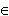R means, that an element à belongs to a set R , i.e. à is an element of the set R . Otherwise, if à doesn't belong to the set R , we write a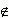R .

Two sets À and B are called equal ( À = Â ), if they consist of the same elements, i.e. each element of the set A is an element of the set B and vice versa, each element of the set Â is an element of the set A .

We say, that a set À is included in a set Â ( Fig.1 ) or the set A is a subset of the set B ( in this case we write À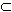Â ), if each element of the set A is an element of the set B . This dependence between sets is called an inclusion . The inclusions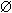À and ÀÀ take place for each set A .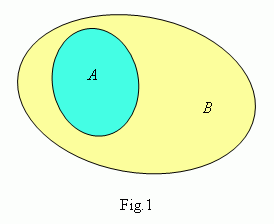A sum ( union ) of sets À and Â ( it's written as À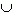Â ) is a set of elements, each of them belongs either to A , or to B . So, åÀÂ , if and only if either åÀ , or åÂ .

A product ( intersection ) of sets À and Â ( it's written as À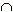Â , Fig.2 ) is a set of elements, each of them belongs both to À and to Â . So, åÀÂ , if and only if åÀ and åÂ .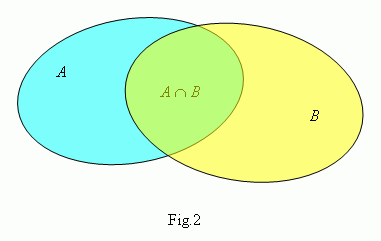A difference of sets À and Â ( it's written as À Â , Fig.3 ) is a set of elements, which belong to the set A , but don't belong to the set Â . This set is called also a complement of the set B relatively the set A .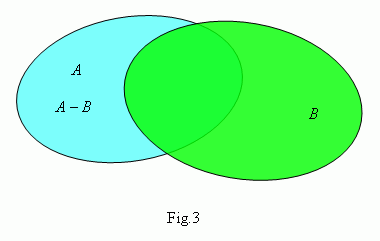A symmetric difference of sets A and B ( it's written as À \ Â ), is called a set:

À \ Â = ( A B )( Â A ) .

Properties of operations with sets: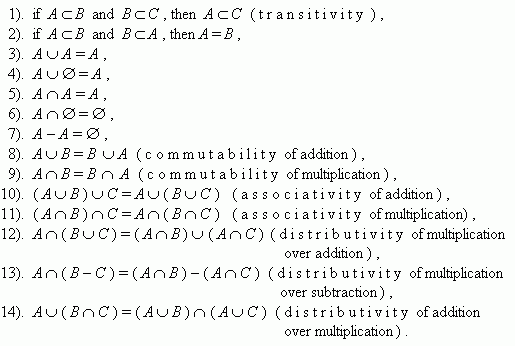E x a m p l e s. 1.  A set of children is a subset of the whole population.

2.  An intersection of the set of integers and the set of positive

numbers is the set of natural numbers.

3.  A union of the set of rational numbers and the set of irrational

numbers is the set of real numbers.

4.  Zero is a complement of the set of natural numbers relatively

the set of non-negative integers.